# NR PUSCH Resource Allocation and DM-RS and PT-RS Reference Signals

This example shows the time-frequency aspects of the new radio (NR) physical uplink shared channel (PUSCH), the associated demodulation reference signal (DM-RS), and phase tracking reference signal (PT-RS). The example shows how PUSCH resource allocation affects the time-frequency structure of DM-RS and PT-RS.

### Introduction

In 5G NR, PUSCH is the physical uplink channel that carries user data. DM-RS and PT-RS are the reference signals associated with PUSCH. DM-RS is used for channel estimation as part of coherent demodulation of PUSCH. To compensate for the common phase error (CPE), 3GPP 5G NR introduced PT-RS. Phase noise produced in local oscillators introduces a significant degradation at mmWave frequencies. It produces CPE and inter-carrier interference (ICI). CPE leads to an identical rotation of a received symbol in each subcarrier. ICI leads to a loss of orthogonality between the subcarriers. PT-RS is used mainly to estimate and minimize the effect of CPE on system performance.

The time-frequency structure of reference signals depends on the type of waveform configured for PUSCH, as defined in TS 38.211 Sections 6.4.1.1 and 6.4.1.2 . When transform precoding is disabled, the waveform configured is cyclic-prefix-orthogonal frequency division multiplexing (CP-OFDM). When transform precoding is enabled, the waveform configured is discrete-fourier-transform-spread orthogonal frequency division multiplexing (DFT-s-OFDM).

The 5G Toolbox™ provides the functions for physical (PHY) layer modeling with varying levels of granularity. The levels of granularity range from PHY channel level functions that perform the transport and physical channel processing to individual channel processing stage functions performing cyclic redundancy check (CRC) coding, code block segmentation, low density parity check (LDPC) channel coding, and so on. The toolbox provides reference signals functionality associated with the PUSCH as functions `nrPUSCHDMRS`, `nrPUSCHDMRSIndices`, `nrPUSCHPTRS`, and `nrPUSCHPTRSIndices`.

### PUSCH

PUSCH is the physical channel that carries the user data. The resources allocated for PUSCH are within the bandwidth part (BWP) of the carrier, as defined in TS 38.214 Section 6.1.2 . The resources in time domain for PUSCH transmission are scheduled by downlink control information (DCI) in the field Time domain resource assignment. This field indicates the slot offset ${\mathit{K}}_{0}$, starting symbol S, the allocation length L, and the mapping type of PUSCH. The valid combinations of S and L are shown in Table 1.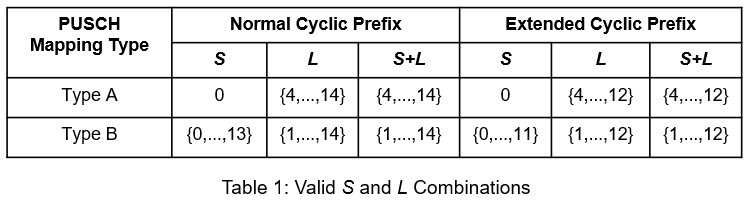The resources in the frequency domain for PUSCH transmission are scheduled by a DCI in the field Frequency domain resource assignment. This field indicates whether the resource allocation of resource blocks (RBs) is contiguous or noncontiguous, based on the allocation type. The RBs allocated are within the BWP.

The 5G Toolbox™ provides the `nrCarrierConfig` and `nrPUSCHConfig` objects to set the parameters related to the PUSCH within the BWP.

```% Setup the carrier with 15 kHz subcarrier spacing and 10 MHz bandwidth carrier = nrCarrierConfig; carrier.SubcarrierSpacing = 15; carrier.CyclicPrefix = 'normal'; carrier.NSizeGrid = 52; carrier.NStartGrid = 0; % Configure the physical uplink shared channel parameters pusch = nrPUSCHConfig; pusch.NSizeBWP = []; % Empty implies that the value is equal to NSizeGrid pusch.NStartBWP = []; % Empty implies that the value is equal to NStartGrid pusch.PRBSet = 0:25; % Allocate half of the carrier bandwidth pusch.SymbolAllocation = [0 14]; % Symbol allocation [S L] pusch.MappingType = 'A'; % PUSCH mapping type ('A' or 'B') pusch.TransmissionScheme = 'nonCodebook'; % ('codebook' or 'nonCodebook') % The following parameters are applicable when TransmissionScheme is set % to 'codebook' pusch.NumAntennaPorts = 4; pusch.TPMI = 0;```

### DM-RS for CP-OFDM

DM-RS is used to estimate the radio channel. DM-RS is present only in the RBs scheduled for PUSCH. The DM-RS structure is designed to support different deployment scenarios and use cases.

#### Parameters That Control Time Resources

The parameters that control the time resources of DM-RS are:

• PUSCH symbol allocation

• Mapping type

• Intra-slot frequency hopping

• DM-RS type A position

• DM-RS length

Symbol allocation of PUSCH indicates the OFDM symbol locations allocated for the PUSCH transmission in a slot. The mapping type indicates the first DM-RS OFDM symbol location and the duration of OFDM symbols (${\mathit{l}}_{\mathit{d}}$). For mapping type A, ${\mathit{l}}_{\mathit{d}}$ is the duration between the first OFDM symbol of the slot and the last OFDM symbol of the allocated PUSCH resources. For mapping type B, ${\mathit{l}}_{\mathit{d}}$ is the duration of the allocated PUSCH resources. When intra-slot frequency hopping is enabled, ${\mathit{l}}_{\mathit{d}}$ is the duration per hop. The DM-RS symbols are present in each hop when intra-slot frequency hopping is enabled. When intra-slot frequency hopping is enabled, DM-RS is single-symbol with the maximum number of additional positions either 0 or 1. The DM-RS symbol locations is given by TS 38.211 Tables 6.4.1.1.3-3, 6.4.1.1.3-4, and 6.4.1.1.3-6. Figure 1 shows the DM-RS symbol locations for PUSCH occupying 14 symbols with PUSCH mapping type A, intra-slot frequency hopping enabled, and number of DM-RS additional positions as 1. The figure shows DM-RS is present in each hop. The locations of DM-RS symbols in each hop depends on the number of OFDM symbols allocated for PUSCH in each hop.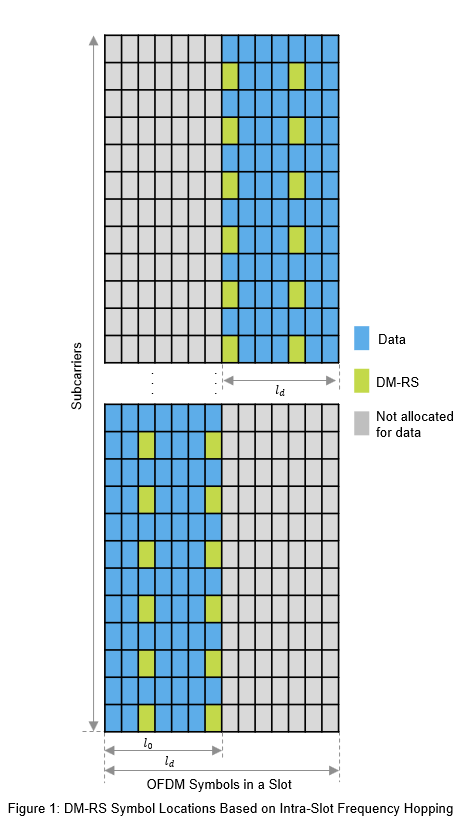For details on other DM-RS parameters, see NR PDSCH Resource Allocation and DM-RS and PT-RS Reference Signals.

```% Assign intra-slot frequency hopping for PUSCH pusch.FrequencyHopping = 'intraSlot'; % 'neither', 'intraSlot', 'interSlot' pusch.SecondHopStartPRB = 26; % Set the parameters that control the time resources of DM-RS pusch.DMRS.DMRSTypeAPosition = 2; % 2 or 3 pusch.DMRS.DMRSLength = 1; % 1 or 2 (single-symbol or double-symbol) pusch.DMRS.DMRSAdditionalPosition = 1; % 0...3 (Number of additional DM-RS positions)```

#### Parameters That Control Frequency Resources

The parameters that control the frequency resources of DM-RS are:

• DM-RS configuration type

• DM-RS antenna ports

The configuration type indicates the frequency density of DM-RS and is signaled by the RRC message dmrs-Type. Configuration type 1 defines six subcarriers per physical resource block (PRB) per antenna port, comprising alternate subcarriers. Configuration type 2 defines four subcarriers per PRB per antenna port, consisting of two groups of two consecutive subcarriers. Different delta shifts are applied to the sets of subcarriers used, depending on the associated antenna port or code division multiplexing (CDM) group. For configuration type 1, there are two possible CDM groups/shifts across eight possible antenna ports (p=0...7). For configuration type 2, there are three possible CDM groups/shifts across twelve antenna ports (p=0...11). For more details, see NR PDSCH Resource Allocation and DM-RS and PT-RS Reference Signals.

In the case of codebook-based PUSCH processing, the union of DM-RS subcarrier locations present in each layer are projected to all the antenna ports.

```% Set the parameters that control the frequency resources of DM-RS pusch.DMRS.DMRSConfigurationType = 1; % 1 or 2 pusch.DMRS.DMRSPortSet = 0; % The read-only properties DeltaShifts and DMRSSubcarrierLocations of DMRS % property of pusch object provides the values of delta shift(s) and DM-RS % subcarrier locations in an RB for each antenna port configured. pusch.DMRS.DeltaShifts```
```ans = 0 ```
`pusch.DMRS.DMRSSubcarrierLocations`
```ans = 6×1 0 2 4 6 8 10 ```

#### Sequence Generation

The pseudorandom sequence used for DM-RS is ${2}^{31}-1$length gold sequence. The sequence is generated across all the common resource blocks (CRBs) and is transmitted only in the RBs allocated for data because the sequence is not required to estimate the channel outside the frequency region in which data is not transmitted. Generating the reference signal sequence across all the CRBs ensures that the same underlying pseudorandom sequence is used for multiple UEs on overlapping time-frequency resources in the case of a multi-user MIMO. The parameters that control the sequence generation are:

• DM-RS scrambling identity (${\mathit{N}}_{\mathrm{ID}}^{{\mathit{n}}_{\mathrm{SCID}}}$)

• DM-RS scrambling initialization (${\mathit{n}}_{\mathrm{SCID}}$)

• Number of OFDM symbols in a slot

• Slot number in a radio frame

• DM-RS symbol locations

• PRBs allocation

The CyclicPrefix property of the carrier object controls the number of OFDM symbols in a slot. The NSlot property of the carrier object controls the slot number.

In the case of codebook-based PUSCH processing, the sequence is multiplied with a precoder matrix, which depends on the number of layers, number of antenna ports, and the transmitted precoder matrix indicator (TPMI).

```% Set the parameters that only control the DM-RS sequence generation pusch.DMRS.NIDNSCID = 1; % Use empty to set it to NCellID of the carrier pusch.DMRS.NSCID = 0; % 0 or 1 % Generate DM-RS symbols pusch.NumLayers = numel(pusch.DMRS.DMRSPortSet); dmrsSymbols = nrPUSCHDMRS(carrier,pusch); % Plot the constellation scatterplot(dmrsSymbols) title('DM-RS Constellation') xlabel('Real') ylabel('Imaginary')``````% The read-only properties TimeWeights and FrequencyWeights of DMRS % property of pusch object provides the values of time and frequency % weights applied to the DM-RS symbols. pusch.DMRS.TimeWeights```
```ans = 2×1 1 1 ```
`pusch.DMRS.FrequencyWeights`
```ans = 2×1 1 1 ```
```% Generate DM-RS indices dmrsIndices = nrPUSCHDMRSIndices(carrier,pusch); % Map the DM-RS symbols to the grid with the help of DM-RS indices if strcmpi(pusch.TransmissionScheme,'codebook') nports = pusch.NumAntennaPorts; else nports = pusch.NumLayers; end grid = zeros([12*carrier.NSizeGrid carrier.SymbolsPerSlot nports]); grid(dmrsIndices) = dmrsSymbols; figure imagesc(abs(grid(:,:,1))); axis xy; xlabel('OFDM Symbols'); ylabel('Subcarriers'); title('DM-RS Time-Frequency Locations');```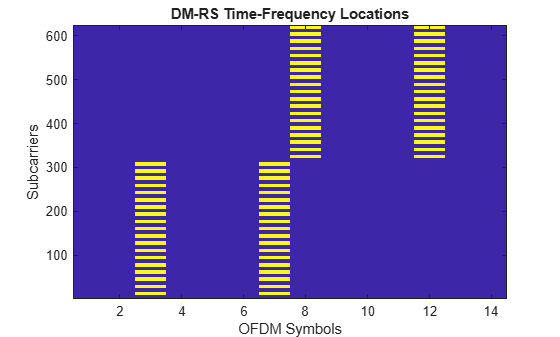### PT-RS for CP-OFDM

PT-RS is the phase tracking reference signal. PT-RS is used mainly to estimate and minimize the effect of CPE on system performance. Due to the phase noise properties, the PT-RS signal has low density in the frequency domain and high density in the time domain. PT-RS always occurs in combination with DM-RS and only when the network has configured PT-RS to be present.

#### Parameters That Control Time Resources

PT-RS is configured through the higher layer parameter DMRS-UplinkConfig for uplink. The parameters that control the time resources of PT-RS are:

• DM-RS symbol locations

• Time density of PT-RS (${\mathit{L}}_{\mathrm{PT}-\mathrm{RS}}$)

${\mathit{L}}_{\mathrm{PT}-\mathrm{RS}}$ depends on the scheduled modulation and coding scheme. The value must be one of {1, 2, 4}. For the parameters that control DM-RS symbol locations, refer to Parameters that Control DM-RS Time Resources (CP-OFDM).

```% Set the EnablePTRS property in pusch to 1 pusch.EnablePTRS = 1; % Set the parameters that control the time resources of PT-RS pusch.PTRS.TimeDensity = 2;```

#### Parameters That Control Frequency Resources

PT-RS occupies only one subcarrier in an RB for one OFDM symbol. The parameters that control the frequency resources of PT-RS are:

• PRB allocation

• DM-RS configuration type

• Frequency density of PT-RS (${\mathit{K}}_{\mathrm{PT}-\mathrm{RS}}$)

• Radio network temporary identifier (${\mathit{n}}_{\mathrm{RNTI}}$)

• Resource element offset

• PT-RS antenna ports

${\mathit{K}}_{\mathrm{PT}-\mathrm{RS}}$ depends on the scheduled bandwidth. The value is either 2 or 4. The value indicates whether PT-RS is present in every two RBs or every four RBs.

For more details, see NR PDSCH Resource Allocation and DM-RS and PT-RS Reference Signals.

```% Set the parameters that control PT-RS subcarrier locations pusch.RNTI = 1; pusch.DMRS.DMRSConfigurationType = 1; pusch.DMRS.DMRSPortSet = 0; % Set the PT-RS parameters pusch.PTRS.FrequencyDensity = 2; % 2 or 4 pusch.PTRS.REOffset = '10'; % '00', '01', '10', '11' pusch.PTRS.PTRSPortSet = min(pusch.DMRS.DMRSPortSet);```

#### Sequence Generation

The sequence used for generating PT-RS is the same pseudorandom sequence used for the DM-RS sequence generation. In the absence of intra-slot frequency hopping, the values of PT-RS sequence depend on the first DM-RS symbol position. In the presence of intra-slot frequency hopping, the values of PT-RS sequence depend on first DM-RS symbol positions in each hop. For more details, refer the section DM-RS Sequence Generation (CP-OFDM).

In the case of codebook-based PUSCH processing, the sequence is multiplied with a precoder matrix, which depends on the number of layers, number of antenna ports, and the transmitted precoder matrix indicator (TPMI).

```% Set the parameters that control the PT-RS sequence generation pusch.DMRS.NIDNSCID = 1; % Use empty to set it to NCellID of the carrier pusch.DMRS.NSCID = 0; % 0 or 1```

Generate the resource element (RE) indices of PUSCH, DM-RS, and PT-RS. Also, generate DM-RS and PT-RS symbols.

```% Control the resource elements available for data in DM-RS OFDM symbol % locations pusch.DMRS.NumCDMGroupsWithoutData = 1; % PUSCH, DM-RS and PT-RS indices pusch.NumLayers = numel(pusch.DMRS.DMRSPortSet); [puschIndices, puschInfo] = nrPUSCHIndices(carrier,pusch); dmrsIndices = nrPUSCHDMRSIndices(carrier,pusch); ptrsIndices = nrPUSCHPTRSIndices(carrier,pusch); % DM-RS and PT-RS symbols dmrsSymbols = nrPUSCHDMRS(carrier,pusch); ptrsSymbols = nrPUSCHPTRS(carrier,pusch);```

Map PUSCH, DM-RS, and PT-RS RE indices to the grid with scaled values to visualize the respective locations on the grid.

```chpLevel = struct; chpLevel.PUSCH = 0.4; chpLevel.DMRS = 1; chpLevel.PTRS = 1.4; gridCPOFDM = complex(zeros([carrier.NSizeGrid*12 carrier.SymbolsPerSlot nports])); gridCPOFDM(puschIndices) = chpLevel.PUSCH; dmrsFactor = chpLevel.DMRS*(1/(max(abs(dmrsSymbols)))); gridCPOFDM(dmrsIndices) = dmrsFactor*dmrsSymbols; ptrsFactor = chpLevel.PTRS*(1/(max(abs(ptrsSymbols)))); gridCPOFDM(ptrsIndices) = ptrsFactor*ptrsSymbols; plotGrid(gridCPOFDM,1,chpLevel)```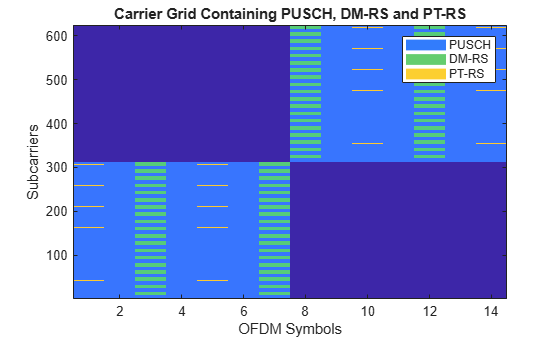In the preceding figure, PT-RS is located from the start of the OFDM symbol in the physical uplink shared channel allocation. The symbols are present at every ${\mathit{L}}_{\mathrm{PT}-\mathrm{RS}}$ hop interval from each other or from DM-RS symbols. The difference in consecutive subcarrier locations of PT-RS is 24, which is the number of subcarriers in an RB (12) times the frequency density of PT-RS (2).

### DM-RS for DFT-s-OFDM

DFT-s-OFDM supports only single layer transmission and is primarily used for low coverage scenarios. The time-frequency resources of DM-RS in DFT-s-OFDM are structured in a way to achieve low cubic metric and high power amplifier efficiency. The transmission of a reference signal frequency multiplexed with other uplink data transmissions highly impacts the power amplifier efficiency due to the increased cubic metric. The reference signals are time-multiplexed with uplink transmissions, thereby blocking all the resource elements for data transmission in the OFDM symbols carrying DM-RS.

#### Parameters That Control Time Resources

The parameters that control the time resources of DM-RS in DFT-s-OFDM are:

• PUSCH symbol allocation

• Mapping type

• Intra-slot frequency hopping

• DM-RS type A position

• DM-RS length

These parameters are the same parameters that control the time resources of DM-RS in CP-OFDM. For more details, refer to Parameters that Control DM-RS Time Resources (CP-OFDM).

```% Set the TransformPrecoding property in pusch to 1 pusch.TransformPrecoding = 1; % Parameters that control the time resources pusch.DMRS.DMRSTypeAPosition = 2; pusch.DMRS.DMRSLength = 1; pusch.DMRS.DMRSAdditionalPosition = 0;```

#### Parameters That Control Frequency Resources

The parameters that control the frequency resources of DM-RS in DFT-s-OFDM are:

• DM-RS configuration type

• DM-RS antenna port

These two parameters are the same as the parameters of CP-OFDM. The DM-RS configuration type is always set to 1. The DM-RS antenna port is nominally a scalar with value 0.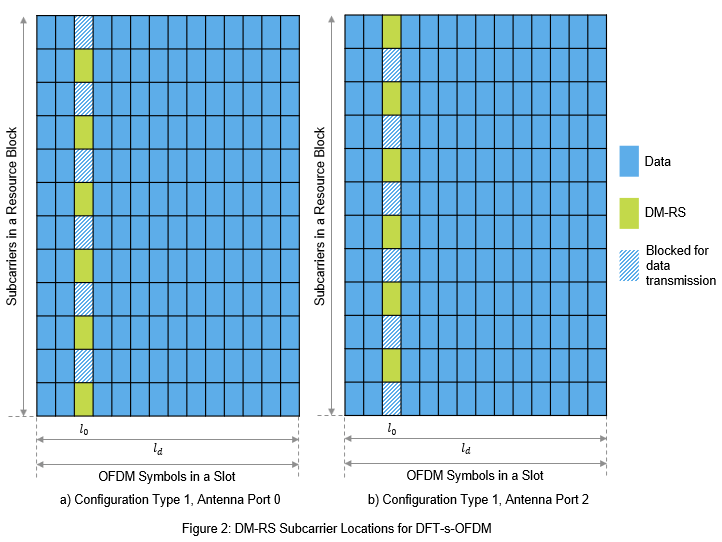There is no need to support multi-user MIMO situations because DFT-s-OFDM is for coverage-limited scenarios. With no MIMO situations, the reference signal is generated only for the transmitted PRBs rather than CRBs as in OFDM. Due to the single layer and single configuration type allowed in DFT-s-OFDM, the number of subcarrier locations used for DM-RS in an RB is constant. Figure 2 illustrates the DM-RS subcarrier locations in DFT-s-OFDM for mapping type A with OFDM symbols allocated for PUSCH spanning over the complete slot.

```% Set the DM-RS antenna port pusch.DMRS.DMRSPortSet = 0;```

#### Sequence Generation

The DM-RS sequence is the ZadoffChu sequence in DFT-s-OFDM. The orthogonal sequences are generated with different cyclic shifts for a group number and sequence number. The parameters that control the sequence generation are:

• PRB allocation

• Group hopping

• Sequence hopping

• DM-RS scrambling identity (${\mathit{N}}_{\mathrm{ID}}^{\mathrm{RS}}$)

• DM-RS symbol locations

```% Parameters that control the sequence generation pusch.DMRS.SequenceHopping = 0; % Sequence hopping (0 or 1) pusch.DMRS.GroupHopping = 1; % Group hopping (0 or 1) pusch.DMRS.NRSID = 1; % Use empty to set it to NCellID of carrier % Generate the DM-RS symbols and indices pusch.NumLayers = numel(pusch.DMRS.DMRSPortSet); dmrsSymbols = nrPUSCHDMRS(carrier,pusch); dmrsIndices = nrPUSCHDMRSIndices(carrier,pusch); dmrsFactor = chpLevel.DMRS*(1/(max(abs(dmrsSymbols)))); % Map DM-RS onto the grid grid = complex(zeros([12*carrier.NSizeGrid carrier.SymbolsPerSlot nports])); grid(dmrsIndices) = dmrsFactor*dmrsSymbols; % Generate PUSCH indices and map onto the grid puschIndices = nrPUSCHIndices(carrier,pusch); grid(puschIndices) = chpLevel.PUSCH; % Plot the grid titleText = 'Carrier Grid Containing PUSCH and DM-RS'; plotGrid(grid,1,struct('PUSCH',chpLevel.PUSCH,'DMRS',chpLevel.DMRS),titleText,{'PUSCH','DM-RS'})```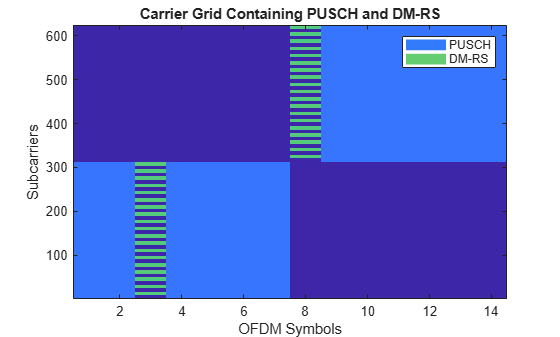The subcarrier locations in the OFDM symbols occupying DM-RS are not allocated for PUSCH.

### PT-RS for DFT-s-OFDM

PT-RS in DFT-s-OFDM is inserted with data in the transform precoding stage.

#### Parameters That Control Time Resources

The parameters that control the time resources of PT-RS in DFT-s-OFDM are same as the parameters that control the time resources of PT-RS in CP-OFDM. The value of ${\mathit{L}}_{\mathrm{PT}-\mathrm{RS}}$ is either 1 or 2 in DFT-s-OFDM. For more details, refer to Parameters that Control PT-RS Time Resources (CP-OFDM).

```% Generate a grid with shared channel allocation for an RB in a single slot % with complete symbol allocation of 14 symbols for a single layer % Set the carrier resource grid with one RB carrier.NSizeGrid = 1; % Configure PUSCH with DFT-s-OFDM and no frequency hopping pusch.TransformPrecoding = 1; pusch.FrequencyHopping = 'neither'; % Set the parameter that control PT-RS time resources pusch.EnablePTRS = 1; pusch.PTRS.TimeDensity = 2;```

#### Parameters That Control Frequency Resources

The PT-RS pattern in the frequency domain is quite different from CP-OFDM. The PT-RS samples are inserted as chunks or groups (${\mathit{N}}_{\mathrm{group}}^{\mathrm{PT}-\mathrm{RS}}$). Each group consists of a finite number of samples (${\mathit{N}}_{\mathrm{samp}}^{\mathrm{group}}$) in the scheduled bandwidth for each OFDM symbol where PT-RS is present.

The parameters that control the frequency resources of PT-RS in DFT-s-OFDM are:

• PRB allocation

• Number of PT-RS samples in a group (${\mathit{N}}_{\mathrm{samp}}^{\mathrm{group}}$)

• Number of PT-RS groups (${\mathit{N}}_{\mathrm{group}}^{\mathrm{PT}-\mathrm{RS}}$)

The valid combinations of PT-RS sample density ([${\mathit{N}}_{\mathrm{samp}}^{\mathrm{group}}$${\mathit{N}}_{\mathrm{group}}^{\mathrm{PT}-\mathrm{RS}}$]) are {[2 2], [2 4], [4 2], [4 4], [4 8]}. The number of PT-RS samples in an OFDM symbol is fixed in DFT-s-OFDM, based on the number of PT-RS samples in all the PT-RS groups. This number is different from CP-OFDM in which the number of PT-RS samples increase based on the number of RBs in PUSCH.

Figure 3 shows the subcarrier locations of PT-RS symbols for an RB with the number of PT-RS samples set to 2 and the number of PT-RS groups set to 2 for an OFDM symbol carrying PT-RS.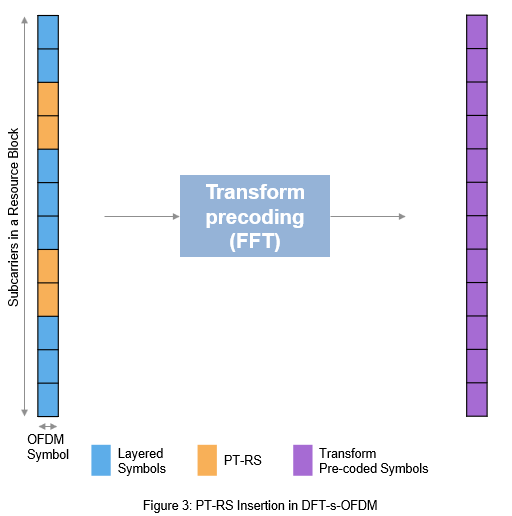PT-RS sample density [2 2] implies that there are two PT-RS groups in a scheduled bandwidth with two symbols each.

PT-RS is inserted with layered symbols at the input of transform precoding. After transform precoding, both layered symbols and PT-RS are treated as data. Therefore, the PT-RS is not visible in the grid directly.

```% Set the parameters that control PT-RS frequency resources pusch.PRBSet = 0:carrier.NSizeGrid-1; pusch.PTRS.NumPTRSSamples = 2; % 2, 4 pusch.PTRS.NumPTRSGroups = 2; % 2, 4, 8```

#### Sequence Generation

The PT-RS sequence in DFT-s-OFDM is a modified pi/2-BPSK sequence. The parameters that control the sequence generation are:

• Starting OFDM symbol of PUSCH allocation

• Number of OFDM symbols in a slot

• Slot number in a radio frame

• PT-RS scrambling identity (${\mathit{N}}_{\mathrm{ID}}$)

• PT-RS subcarrier locations

```% Set the parameters that control PT-RS sequence generation pusch.DMRS.NRSID = 1; pusch.PTRS.NID = 10; % Use empty to set it to NRSID of DMRS configuration```

Generate PUSCH and PT-RS RE indices.

```% PUSCH, and PT-RS indices [puschIndices, puschInfoDFTsOFDM] = nrPUSCHIndices(carrier,pusch); ptrsIndices = nrPUSCHPTRSIndices(carrier,pusch);```

Set PUSCH and PT-RS resource elements to constant values.

```% Insert PT-RS along with the PUSCH data GdPTRS = size(reshape(ptrsIndices,[],pusch.NumLayers),1); dataWithPTRS = chpLevel.PUSCH*ones(puschInfoDFTsOFDM.Gd+GdPTRS,1); dataWithPTRS(ptrsIndices(:,1)) = chpLevel.PTRS;```

Plot PT-RS projections onto the grid.

```gridDFTsOFDM = zeros(numel(pusch.PRBSet)*12, carrier.SymbolsPerSlot); % Map the grid with data and reference signals gridDFTsOFDM(:,puschInfoDFTsOFDM.DMRSSymbolSet+1) = chpLevel.DMRS; gridDFTsOFDM(~(gridDFTsOFDM==chpLevel.DMRS)) = dataWithPTRS; % Plot the projections of data, DM-RS and PT-RS on grid before transform % precoding fNames = {'PUSCH','DM-RS+Res','PT-RS'}; titleText = 'Projection of Data, DM-RS, and PT-RS before Transform Precoding'; plotGrid(gridDFTsOFDM,1,chpLevel,titleText,fNames)```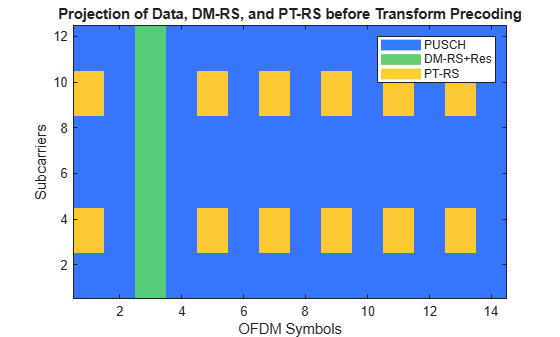### Further Exploration

You can try changing the parameters that affect the time and frequency resources of reference signals and observe the variations in the RE positions for the respective signals.

Try changing the number of antenna ports configured for DM-RS and PT-RS, then observe the variations of reference signals and data across the ports. For example, try to configure DM-RS for two antenna ports 0 and 2, configuration type 1, and PT-RS for antenna port 0. Generate the PUSCH indices, DM-RS signal (indices and symbols), and PT-RS signal (indices and symbols). Map them to a grid and visualize the grid for both the ports.

Try performing channel estimation and phase tracking using the PT-RS symbols and indices. Compute the throughput by following the steps outlined in NR PUSCH Throughput.

This example shows how to generate the DM-RS and PT-RS sequences and how to map the sequences to the OFDM carrier resource grid. It highlights the properties that control the time-frequency structure of reference signals for different waveforms. For example, the time-frequency pattern for reference signals in CP-OFDM and DFT-s-OFDM and the variation in the sequences generated for reference signals in different waveforms.

### References

1. 3GPP TS 38.211. "NR; Physical channels and modulation" 3rd Generation Partnership Project; Technical Specification Group Radio Access Network.

2. 3GPP TS 38.214. "NR; Physical layer procedures for data" 3rd Generation Partnership Project; Technical Specification Group Radio Access Network.

3. 3GPP TS 38.212. "NR; Multiplexing and channel coding" 3rd Generation Partnership Project; Technical Specification Group Radio Access Network.

### Local Functions

```function plotGrid(grid,nLayer,chpLevel,titleText,names) % plotGrid Display resource grid GRID of the layer number NLAYER with the % legend containing physical channel and associated reference signals, at % different power levels CHPLEVEL with title TITLETEXT. Legend is created % using a cell array of character vectors NAMES. if nargin < 4 titleText = 'Carrier Grid Containing PUSCH, DM-RS and PT-RS'; end if nargin < 5 names = {'PUSCH', 'DM-RS', 'PT-RS'}; end map = parula(64); cscaling = 40; im = image(1:size(grid,2),1:size(grid,1),cscaling*abs(grid(:,:,nLayer))); colormap(im.Parent,map); % Add legend to the image chpval = struct2cell(chpLevel); clevels = cscaling*[chpval{:}]; N = length(clevels); L = line(ones(N),ones(N), 'LineWidth',8); % Generate lines % Index the color map and associated the selected colors with the lines set(L,{'color'},mat2cell(map( min(1+clevels,length(map) ),:),ones(1,N),3)); % Set the colors according to map % Create legend legend(names{:}); axis xy; ylabel('Subcarriers'); xlabel('OFDM Symbols'); title(titleText); end```